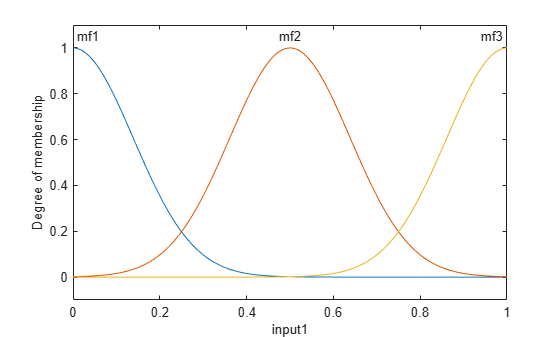Add input variable to fuzzy inference system

## Syntax

``fisOut = addInput(fisIn)``
``fisOut = addInput(fisIn,range)``
``fisOut = addInput(___,Name,Value)``

## Description

example

````fisOut = addInput(fisIn)` adds a default input variable to `fisIn` and returns the resulting fuzzy system in `fisOut`. This input variable has a default name, default range, and no membership functions.```
````fisOut = addInput(fisIn,range)` adds an input variable with the specified range.```

example

````fisOut = addInput(___,Name,Value)`configures the input variable using one or more name-value pair arguments.```

## Examples

collapse all

Create a Sugeno fuzzy inference system.

`fis = sugfis('Name','tipper');`

Add an input variable with default specifications.

`fis = addInput(fis);`

You can configure the input variable properties using dot notation. For example, specify the name and range for the variable.

```fis.Inputs(1).Name = "service"; fis.Inputs(1).Range = [0 10];```

View the input variable.

`fis.Inputs(1)`
```ans = fisvar with properties: Name: "service" Range: [0 10] MembershipFunctions: [0x0 fismf] ```

You can also specify a variable name and range when you add it to the fuzzy system.

```fis2 = sugfis('Name','tipper'); fis2 = addInput(fis2,[0 10],'Name',"service");```

Create a fuzzy inference system.

`fis = mamfis('Name',"tipper");`

Add an input variable with three Gaussian membership functions distributed over the input range.

`fis = addInput(fis,'NumMFs',3,'MFType',"gaussmf");`

View the membership functions.

`plotmf(fis,'input',1)`## Input Arguments

collapse all

Fuzzy inference system, specified as one of the following:

Variable range, specified as a two-element element vector where the first element is less than the second element. The first element specifies the lower bound of the range, and the second element specifies the upper bound of the range.

### Name-Value Pair Arguments

Specify optional comma-separated pairs of `Name,Value` arguments. `Name` is the argument name and `Value` is the corresponding value. `Name` must appear inside quotes. You can specify several name and value pair arguments in any order as `Name1,Value1,...,NameN,ValueN`.

Example: `'NumMFs',3` configures the variable to use three membership functions

Variable name, specified as the comma-separated pair consisting of `'Name'` and a string or character vector. The default variable name is `"input<uniqueIndex>"`, where `uniqueIndex` is automatically generated based on the current number of inputs in `fisIn`.

Number of membership functions, specified as the comma-separated pair consisting of `'NumMFs'` and a nonnegative integer.

Membership function type, specified as the comma-separated pair consisting of `'MFType'` and one of the following:

• `"trimf"` — Triangular membership functions

• `"gaussmf"` — Gaussian membership functions

The membership functions are uniformly distributed over the input variable range with approximately 80% overlap in the membership function supports.

## Output Arguments

collapse all

Fuzzy inference system, specified as one of the following:

• `mamfis` object — Mamdani fuzzy inference system

• `sugfis` object — Sugeno fuzzy inference system

• `mamfistype2` object — Type-2 Mamdani fuzzy inference system

• `sugfistype2` object — Type-2 Sugeno fuzzy inference system

`fisOut` contains the added input variable, with all other properties matching the properties of `fisIn`.